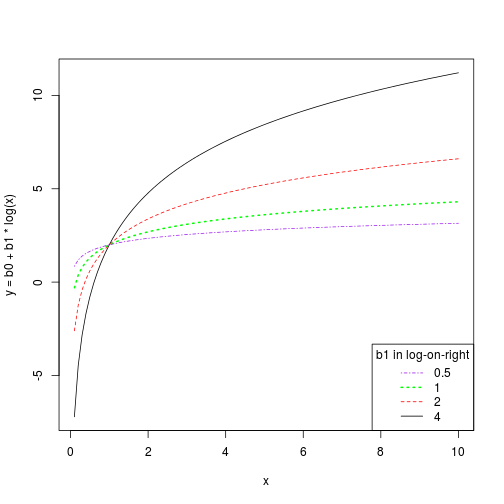## Paul Johnson
## 2013-04-01
## regression-log-on-right-1.R

## If  you fit

## m1 <- lm(y ~ log(x))
##
## how do you understand the coefficient?

## It seems not helpful to most people to note that this predictive
## model
##
## y = b0 + b1 log(x)
##
## is the same as
##
## y = b0 + log(x^b1).
##
##
## So better plot that for various b's to see what it means.

## I'll come back and write more example later, but the immediate need
## right now is to visualize the effect of changing b.

## And its a nice time to test out the curve() function in R,
## which I did not use much in the past because I could not
## understand the manual, but now I do.

curve( 2 + 4 * log(x), from = 0.1, to = 10, ylab = "y = b0 + b1 * log(x)")
curve( 2 + 2 * log(x), from = 0.1, to = 10, add = TRUE, col = "red", lty = 2)
curve( 2 + 1 * log(x), from = 0.1, to = 10, add = TRUE, col = "green", lty = 3, lwd = 2)
curve( 2 + 0.5 * log(x), from = 0.1, to = 10, add = TRUE, col = "purple", lty = 4)
legend("bottomright", legend = c("0.5", "1", "2", "4"),
col = c("purple","green","red", "black"), lty = 4:1,
title = "b1 in log-on-right", lwd = c(1,2,1,1))